# Alarm Clock

In this tutorial you will learn how to create an Analogue Clock User Control which displays the Time and Alarm setting, which can be used to create a simple Alarm Clock!

Step 1

Start Microsoft Visual Basic 2008 Express Edition, then select File then New Project... Choose Windows Forms Application from the New Project Window, enter a name for the Project and then click OK, see below:Step 2

A Blank Form named Form1 should then appear, see below: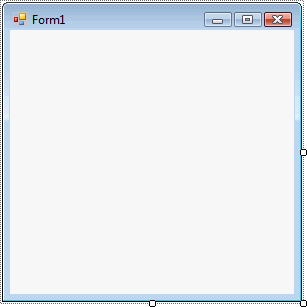Step 3

With Form1 selected goto the Properties box and change the Name from Form1 to frmMain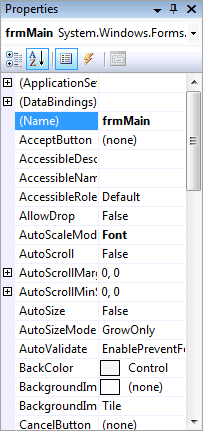Step 4

Then select Project then Add User Control... and choose the "User Control" Template if it is not already selected and give it the Name "Clock.vb" without the quotes, see below:Step 5

With the blank Clock User Control displayed, if not double click on the "Clock.vb" item in Solution Explorer, then right click on the User Control or the entry for it in Solution Explorer, then select View Code, enter the following above the Public Class Clock line:

```Imports System.Drawing.Drawing2D
Imports System.Drawing.Text
```

Then enter the following below the Public Class Clock:

```''' <summary>Tick Style of Clock</summary>
Public Enum TickStyle
Smooth = 0
Normal = 1
End Enum

' Private Members

Private Smooth As SmoothingMode = SmoothingMode.AntiAlias
Private Rendering As TextRenderingHint = TextRenderingHint.AntiAlias
Private tsSecondHand As TickStyle = TickStyle.Normal
Private tsMinuteHand As TickStyle = TickStyle.Normal
Private cSecondHand As Color = Color.Tomato
Private cMinuteHand As Color = Color.Gainsboro
Private cHourHand As Color = Color.WhiteSmoke
Private cAlarmHand As Color = Color.Orange
Private Face As Color = Color.RoyalBlue
Private Rim As Color = Color.Blue
Private NumeralForeColor As Color = Color.WhiteSmoke
Private ClockTime As DateTime = Now()
Private AlarmTime As DateTime
Private NumeralFont As Font = Me.Font
Private DrawSecondHand As Boolean = True
Private DrawMinuteHand As Boolean = True
Private DrawHourHand As Boolean = True
Private DrawAlarmHand As Boolean = True
Private DrawNumerals As Boolean = True
Private midX As Single
Private midY As Single
Private Y As Single
Private X As Single
Private SecondAngle As Single
Private MinuteAngle As Single
Private HourAngle As Single
Private AlarmAngle As Single
Private Hour As Integer
Private Minute As Integer
Private Second As Integer
```

See Below: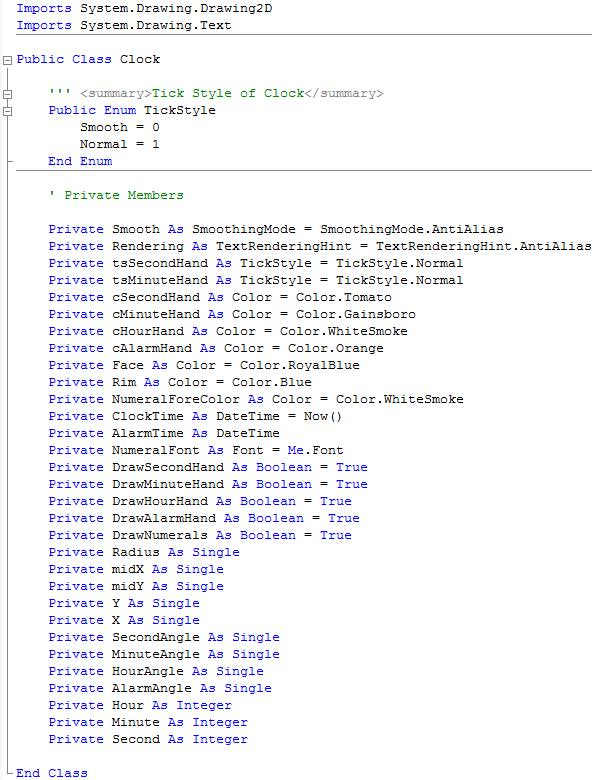Step 6

With the Clock User Control Code View still displayed, enter the following Private Methods below the "Private Members" section and above "End Class":

```' Private Methods

''' <summary>GetX</summary>
''' <param name="Deg">Degrees</param>
Private Function GetX(ByVal Deg As Single)
Return CType(Radius * Math.Cos((Math.PI / 180) * Deg), Single)
End Function

''' <summary>GetY</summary>
''' <param name="Deg">Degrees</param>
Private Function GetY(ByVal Deg As Single)
Return CType(Radius * Math.Sin((Math.PI / 180) * Deg), Single)
End Function

''' <summary>Draw Clock on GDI Surface</summary>
''' <param name="g">Graphics Surface</param>
Private Sub DrawClock(ByVal g As Graphics)
Dim Surface As Graphics = g
Surface.SmoothingMode = Smooth
Surface.TextRenderingHint = Rendering
Surface.InterpolationMode = System.Drawing.Drawing2D.InterpolationMode.HighQualityBicubic
midX = ClientSize.Width / 2
midY = ClientSize.Height / 2
X = ClientSize.Width
Y = ClientSize.Height
Dim TextBrush As New SolidBrush(NumeralForeColor)
Dim Pen As New Pen(NumeralForeColor)
' Define Rectangles
Dim Rect As New Rectangle(0 + 10, 0 + 10, CInt(X - 20), CInt(Y - 20))
Dim RectRim As New Rectangle(0 + 20, 0 + 20, CInt(X - 40), CInt(Y - 40))
Dim RectInner As New Rectangle(0 + 40, 0 + 40, CInt(X - 80), CInt(Y - 80))
NumeralFont = Me.Font
' Face
Dim FaceBrush As New SolidBrush(Face)
Surface.FillEllipse(FaceBrush, Rect)
' Rim
Dim RimBrush = New SolidBrush(Rim)
Surface.FillEllipse(RimBrush, RectRim)
Surface.TranslateTransform(midX, midY) ' Define Centre
Using Format As New StringFormat
Format.Alignment = StringAlignment.Center
Format.LineAlignment = StringAlignment.Center
' Draw Numerals
Dim deg As Integer = 360 / 12
If Numerals Then
For i As Integer = 1 To 12
Surface.DrawString(i.ToString(), NumeralFont, TextBrush, _
-1 * GetX(i * deg + 90), -1 * GetY(i * deg + 90), Format)
Next
End If
End Using
Hour = ClockTime.Hour
Minute = ClockTime.Minute
Dim Centre As New Point(0, 0)
If DrawAlarmHand Then ' Draw Alarm Hand
AlarmAngle = 2.0 * Math.PI * (AlarmTime.Hour + AlarmTime.Minute / 60.0) / 12.0
Pen.EndCap = LineCap.ArrowAnchor
Pen.StartCap = LineCap.RoundAnchor
Pen.Color = cAlarmHand
Dim AlarmHand As New Point(CInt(Radius * Math.Sin(AlarmAngle) / 1.75), _
Surface.DrawLine(Pen, Centre, AlarmHand) ' Alarm Hour
AlarmAngle = CType(2.0 * Math.PI * (AlarmTime.Minute + AlarmTime.Second / 60.0) / 60.0, Single)
AlarmHand = New Point(CInt(Radius * Math.Sin(AlarmAngle) / 1.25), _
Surface.DrawLine(Pen, Centre, AlarmHand) ' Alarm Minute
End If
If DrawHourHand Then ' Draw Hour Hand
HourAngle = 2.0 * Math.PI * (Hour + Minute / 60.0) / 12.0
Pen.EndCap = LineCap.Round
Pen.StartCap = LineCap.RoundAnchor
Pen.Color = cHourHand
Dim HourHand As New Point(CInt(Radius * Math.Sin(HourAngle) / 1.5), _
Surface.DrawLine(Pen, Centre, HourHand)
End If
If DrawMinuteHand Then ' Draw Minute Hand
If tsMinuteHand = TickStyle.Smooth Then
MinuteAngle = CType(2.0 * Math.PI * (Minute + Second / 60.0) / 60.0, Single)
Else
MinuteAngle = CType(2.0 * Math.PI * (Minute / 60.0), Single)
End If
Pen.EndCap = LineCap.Round
Pen.StartCap = LineCap.RoundAnchor
Pen.Color = cMinuteHand
Dim MinHand As New Point(CInt(Radius * Math.Sin(MinuteAngle)), _
Surface.DrawLine(Pen, Centre, MinHand)
End If
If DrawSecondHand Then ' Draw Second Hand
If tsSecondHand = TickStyle.Smooth Then
Second = ClockTime.Second + (ClockTime.Millisecond * 0.001)
Else
Second = ClockTime.Second
End If
SecondAngle = 2.0 * Math.PI * Second / 60.0
Pen.EndCap = LineCap.Triangle
Pen.StartCap = LineCap.RoundAnchor
Pen.Color = cSecondHand
Dim SecHand As New Point(CInt(Radius * Math.Sin(SecondAngle)), _
Surface.DrawLine(Pen, Centre, SecHand)
End If
Pen.Dispose() ' Cleanup Pen
End Sub
```

See Below: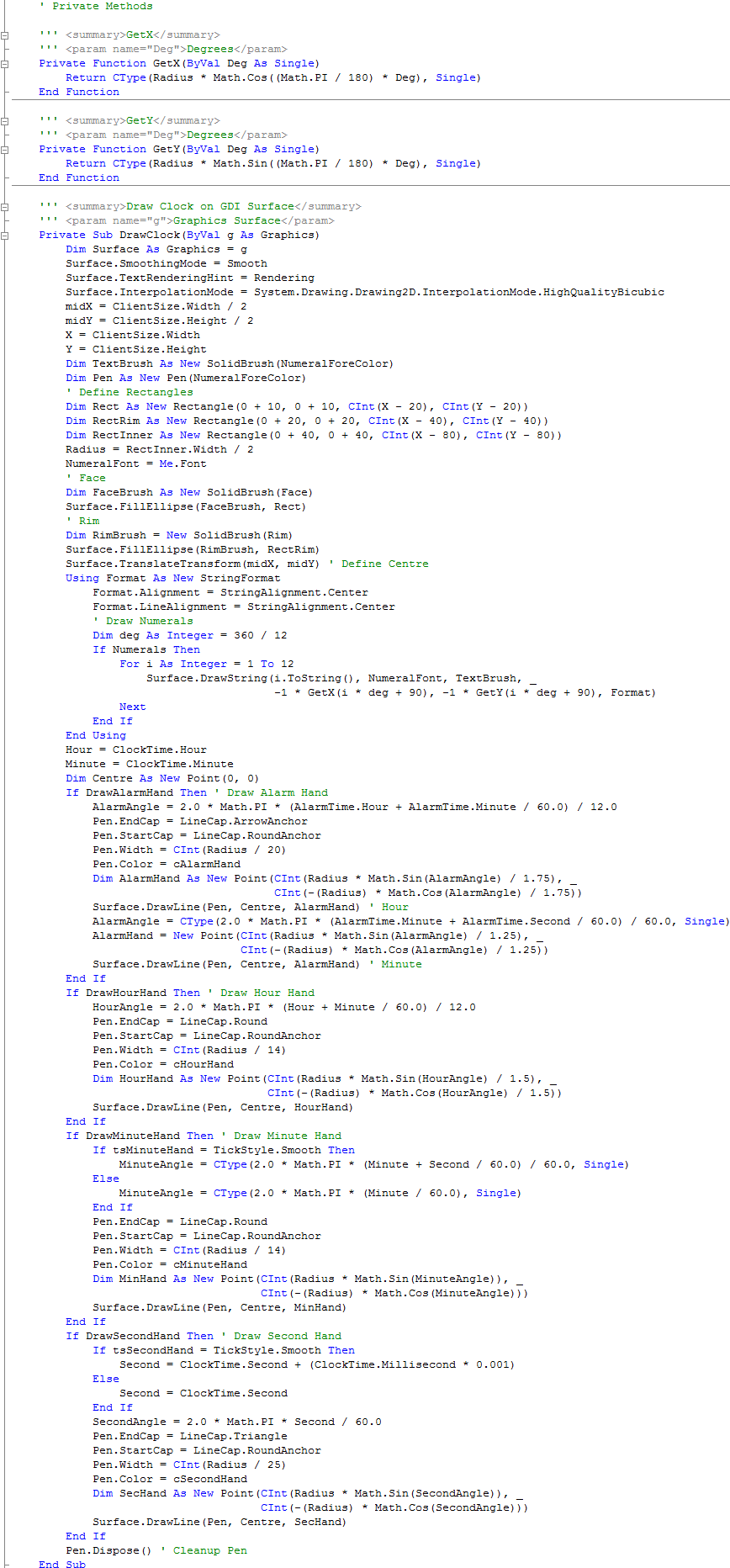Step 7

Again, with the Clock User Control Code View still displayed, enter the Constructor and following Public Properties above "End Class" and below the "Private Methods" section:

```''' <summary>Constructor</summary>
Public Sub New()
' This call is required by the Windows Form Designer.
InitializeComponent()
' Add any initialization after the InitializeComponent() call.
Me.SetStyle(ControlStyles.OptimizedDoubleBuffer, True)
Me.SetStyle(ControlStyles.UserPaint, True)
Me.SetStyle(ControlStyles.AllPaintingInWmPaint, True)
End Sub

' Public Properties

''' <summary>Determines what Draw Smoothing should be applied</summary>
''' <value>SmoothingMode</value>
''' <returns>Smooth as SmoothingMode</returns>
Public Property DrawSmoothing() As SmoothingMode
Get
Return Smooth
End Get
Set(ByVal Value As SmoothingMode)
Smooth = Value
Invalidate()
End Set
End Property

''' <summary>Determines what Text Smoothing should be applied</summary>
''' <value>TextRenderingHint</value>
''' <returns>Rendering as TextRenderingHint</returns>
Public Property TextSmoothing() As TextRenderingHint
Get
Return Rendering
End Get
Set(ByVal Value As TextRenderingHint)
Rendering = Value
Invalidate()
End Set
End Property

''' <summary>Defines Second Hand Tick Style</summary>
''' <value>Value as TickStyle</value>
''' <returns>SecondHand TickStyle</returns>
Public Property SecondHandTickStyle() As TickStyle
Get
Return tsSecondHand
End Get
Set(ByVal Value As TickStyle)
tsSecondHand = Value
Invalidate()
End Set
End Property

''' <summary>Defines Minute Hand Tick Style</summary>
''' <value>Value as TickStyle</value>
''' <returns>MinuteHand TickStyle</returns>
Public Property MinuteHandTickStyle() As TickStyle
Get
Return tsMinuteHand
End Get
Set(ByVal Value As TickStyle)
tsMinuteHand = Value
Invalidate()
End Set
End Property

''' <summary>Clock Face Colour</summary>
Public Property FaceColour() As Color
Get
Return Face
End Get
Set(ByVal Value As Color)
Face = Value
Invalidate()
End Set
End Property

''' <summary>Clock Rim Colour</summary>
Public Property RimColour() As Color
Get
Return Rim
End Get
Set(ByVal Value As Color)
Rim = Value
Invalidate()
End Set
End Property

''' <summary>Second Hand Colour</summary>
Public Property SecondHandColor() As Color
Get
Return cSecondHand
End Get
Set(ByVal Value As Color)
cSecondHand = Value
Invalidate()
End Set
End Property

''' <summary>Minute Hand Colour</summary>
Public Property MinuteHandColor() As Color
Get
Return cMinuteHand
End Get
Set(ByVal Value As Color)
cMinuteHand = Value
Invalidate()
End Set
End Property

''' <summary>Hour Hand Colour</summary>
Public Property HourHandColor() As Color
Get
Return cHourHand
End Get
Set(ByVal Value As Color)
cHourHand = Value
Invalidate()
End Set
End Property

''' <summary>Alarm Hand Colour</summary>
Public Property AlarmHandColor() As Color
Get
Return cAlarmHand
End Get
Set(ByVal Value As Color)
cAlarmHand = Value
Invalidate()
End Set
End Property

''' <summary>Numeral Colour</summary>
Public Property NumeralColour() As Color
Get
Return NumeralForeColor
End Get
Set(ByVal Value As Color)
NumeralForeColor = Value
Invalidate()
End Set
End Property

''' <summary>Draw Second Hand</summary>
Public Property SecondHand() As Boolean
Get
Return DrawSecondHand
End Get
Set(ByVal Value As Boolean)
DrawSecondHand = Value
Invalidate()
End Set
End Property

''' <summary>Draw Minute Hand</summary>
Public Property MinuteHand() As Boolean
Get
Return DrawMinuteHand
End Get
Set(ByVal Value As Boolean)
DrawMinuteHand = Value
Invalidate()
End Set
End Property

''' <summary>Draw Hour Hand</summary>
Public Property HourHand() As Boolean
Get
Return DrawHourHand
End Get
Set(ByVal Value As Boolean)
DrawHourHand = Value
Invalidate()
End Set
End Property

''' <summary>Draw Alarm Hand</summary>
Public Property AlarmHand() As Boolean
Get
Return DrawAlarmHand
End Get
Set(ByVal Value As Boolean)
DrawAlarmHand = Value
Invalidate()
End Set
End Property

''' <summary>Draw Clock Face Numerals</summary>
Public Property Numerals() As Boolean
Get
Return DrawNumerals
End Get
Set(ByVal Value As Boolean)
DrawNumerals = Value
Invalidate()
End Set
End Property

''' <summary>Clock Time</summary>
Public Property Time() As DateTime
Get
Return ClockTime
End Get
Set(ByVal Value As DateTime)
ClockTime = Value
Invalidate()
End Set
End Property

''' <summary>Alarm Time</summary>
Public Property Alarm() As DateTime
Get
Return AlarmTime
End Get
Set(ByVal Value As DateTime)
AlarmTime = Value
Invalidate()
End Set
End Property
```

See Below:Step 8

Again while still in the Code View, there are two drop-down boxes, select "(Clock Events)" from the first "(General)", and then from the other "(Declarations)" select "Paint" and type the following in the Clock_Paint(...) Sub:

```DrawClock(e.Graphics)
```

Finally, select "(Clock Events)" from first drop-down list in Code View again then select "Resize" from the "(Declarations)" drop-down and type the following in the Clock_Resize(...) Sub:

```Me.Width = Me.Height
Me.Height = Me.Width
Invalidate()
```

See Below: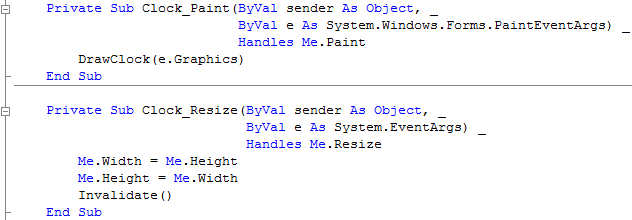Step 9

The Clock User Control is now Completed, to use it in the application click on Build, then "Build AlarmClock":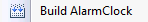You may be asked to Save your Project, when you do and the Build has completed. Select the Clock Component that appears in the Toolbox under AlarmClock Components: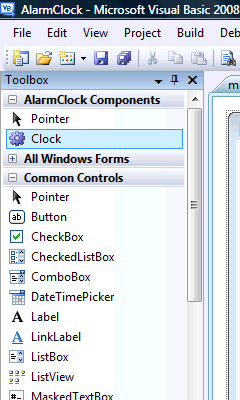Step 10

Display frmMain by double-clicking on the entry for it in Solution Explorer, or select the "frmMain [Design]" Tab, then draw the Clock Component on the Form, make sure to position and size the Control so it appears as below: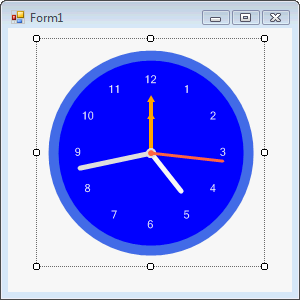Step 11

Then goto the Properties box and change the Name to "Clock", without quotes, see Below:Step 12

Then from the Common Controls tab on the Toolbox select the CheckBox component: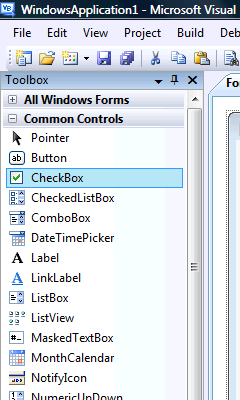Step 13

Draw a CheckBox on the Form, make sure to position the Control so it appears as below:Step 14

Then goto the Properties box and change the Name to "SetAlarm" and Text to "Set Alarm", both without quotes, see Below: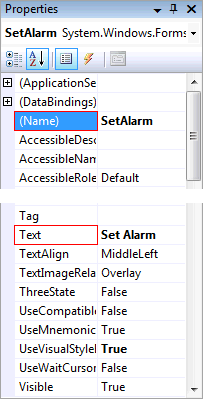Step 15

Then from the Common Controls tab on the Toolbox select the DateTimePicker Control, see Below:Step 16

Draw a DateTimePicker on the Form, make sure to position and size the Control so it appears as below: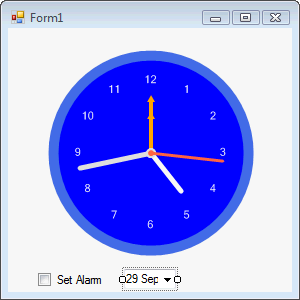Step 17

Then goto the Properties box and change the Name to "Alarm", CustomFormat to "hh:mm", Format to "Custom" and ShowUpDown to "True", all without quotes, see Below: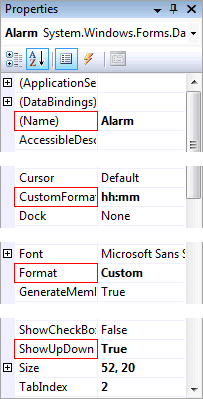Step 18

Then from the Components tab on the Toolbox select the Timer Control, see Below: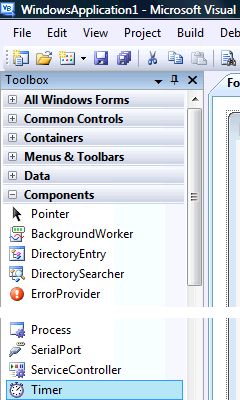Step 19

Draw a Timer on the Form, or double-click on the Timer entry in the Toolbox, and it will appear in the pane, beneath the form, see below:Step 20

Then goto the Properties box and change the Name to "Display, Enabled to "True" and the Interval property to "100", all without quotes, see Below:Step 21

Click on the [Design] Tab to view the form again or double click on the frmMain entry in Solution Explorer, then Double-click on the DateTimePicker, and type the following in the Alarm_ValueChanged(...) Sub:

```Clock.Alarm = Alarm.Value
```

See Below: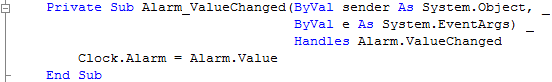Step 22

Click on the [Design] Tab to view the form again or double click on the frmMain entry in Solution Explorer, then Double-click on the Timer called Display in the Pane below frmMain, and type the following in the Display_Tick(...) Sub:

```Clock.Time = Now() ' Update Clock Time
If Format(Clock.Time, "hh:mm:ss") = _
Format(Clock.Alarm, "hh:mm") & ":00" And _
SetAlarm.Checked Then
Display.Stop() ' Stop Clock
MsgBox("Alarm!", MsgBoxStyle.SystemModal, "Alarm Clock")
Display.Start() ' Start Clock
End If
```

See Below: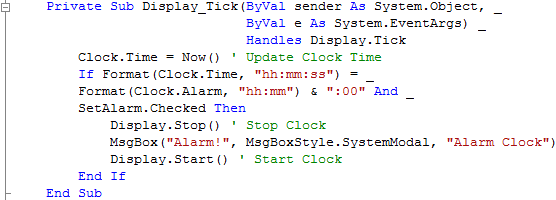Step 23

Click on the [Design] Tab to view the form again, then Double Click on the Form (frmMain) and type the following in the frmMain_Load() Sub

```Clock.Alarm = Now()
Alarm.Value = Now()
Me.Text = "Alarm Clock"
```

See Below: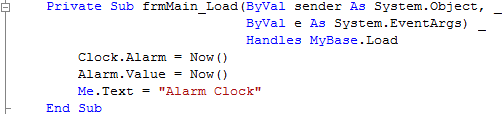Step 24

Save the Project as you have now finished the application, then click on Start:When you do the following will appear: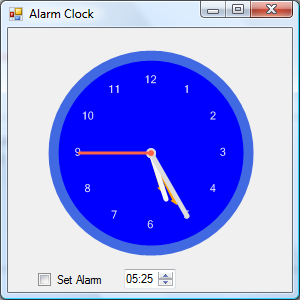Step 25

Set the Alarm using the DateTimePicker then Check the Set Alarm Checkbox, a Message Box will appear when the Alarm goes off, see below: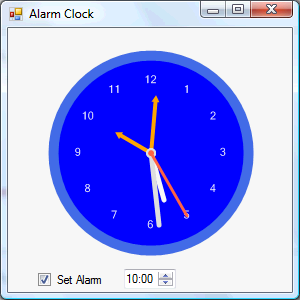Step 26

Click on the Close button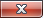on the top right to end the application.

This is a very simple Alarm Clock application, you can enhance the Clock by using different colours or change SolidBrush to something else such as a GradientBrush! You can also improve what happens when the Alarm goes off, it is up to you, make it your own!